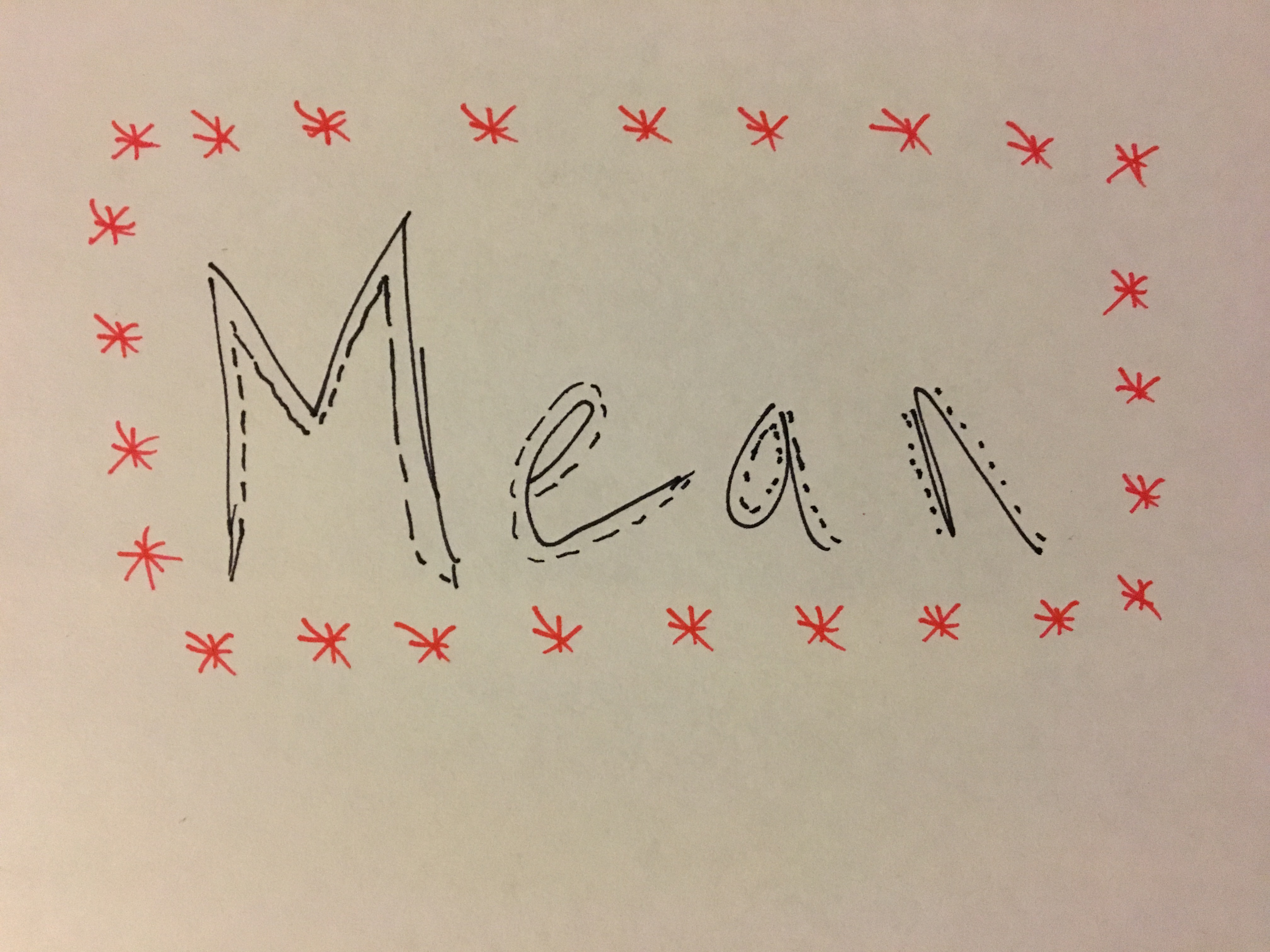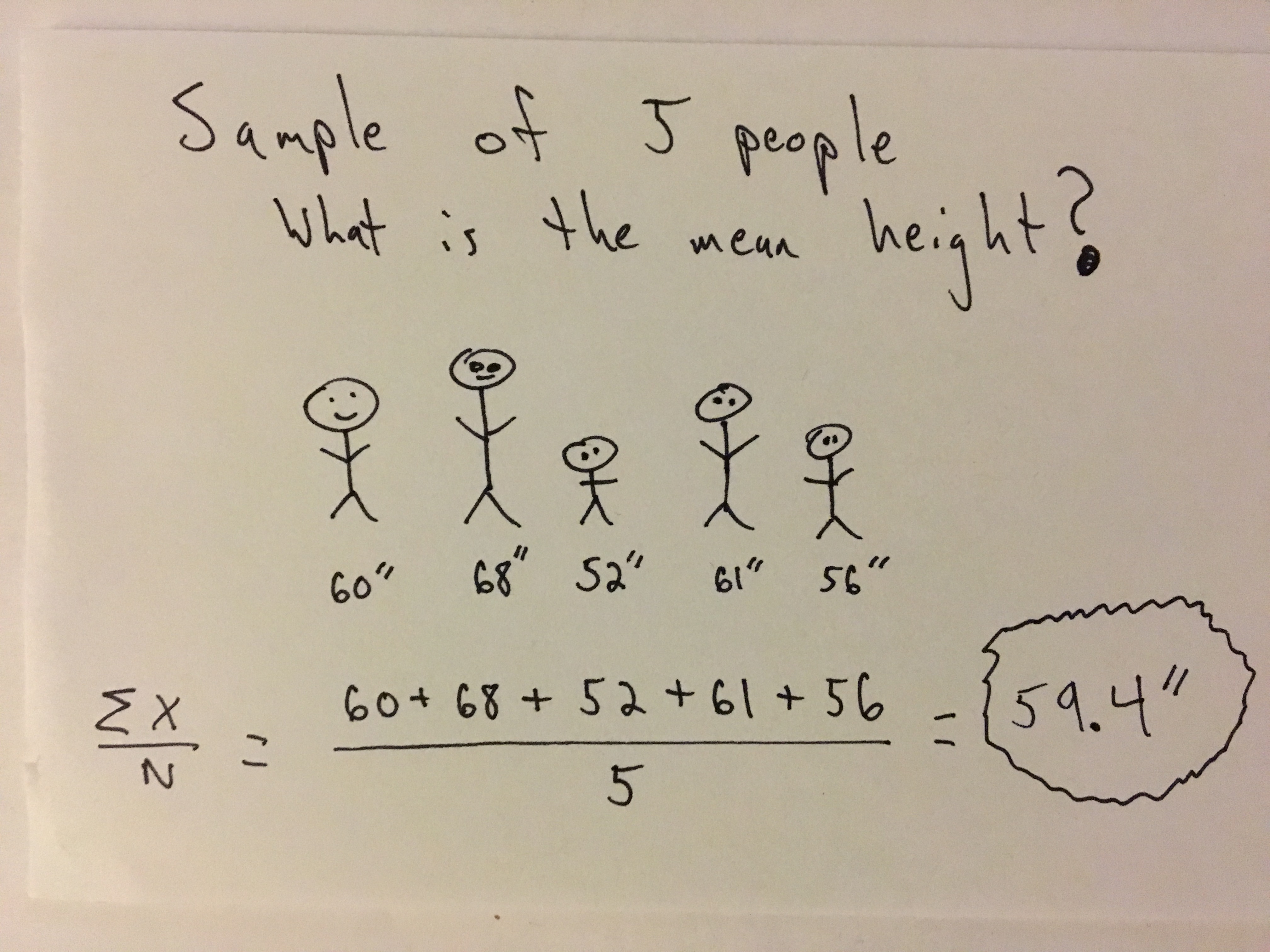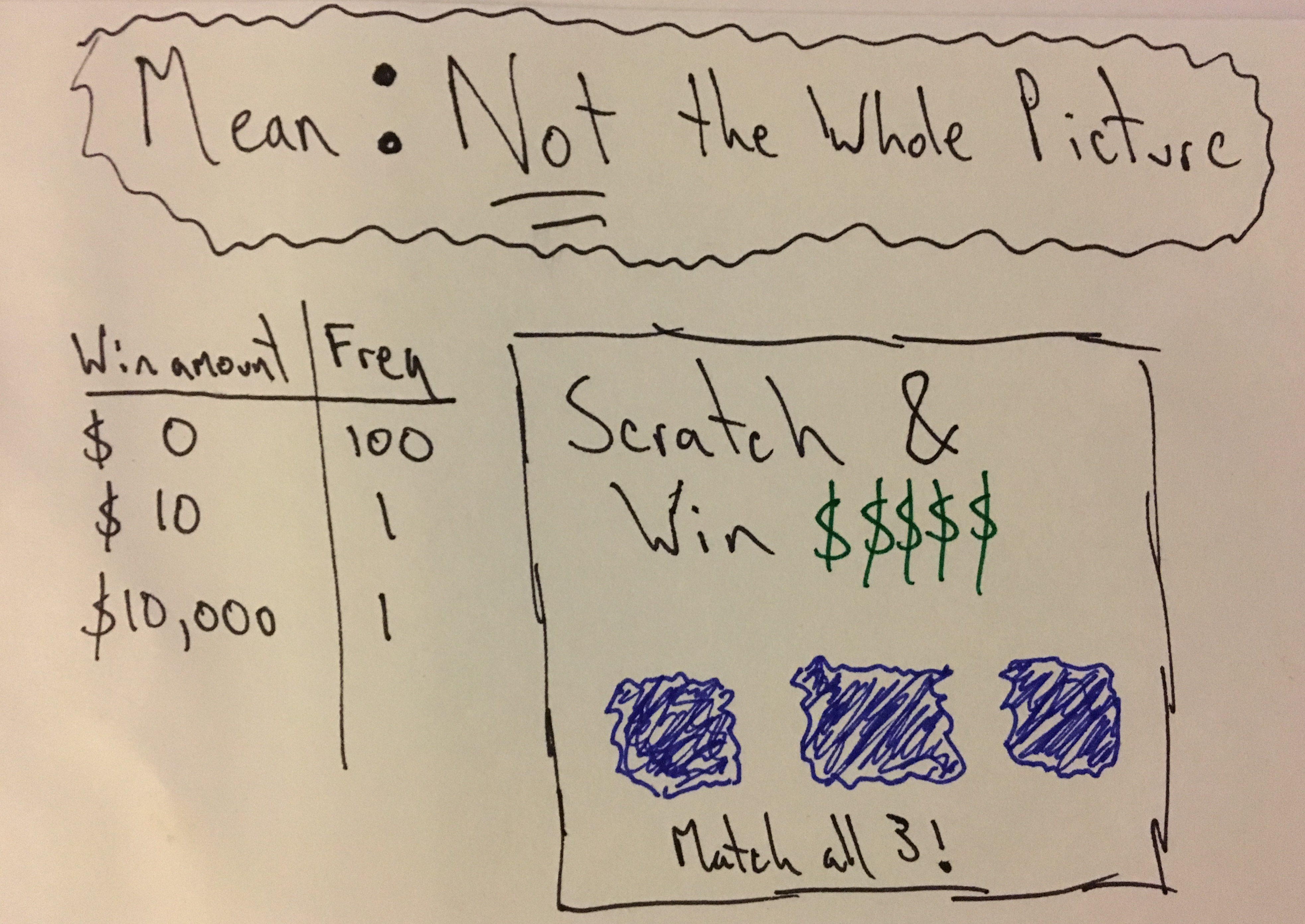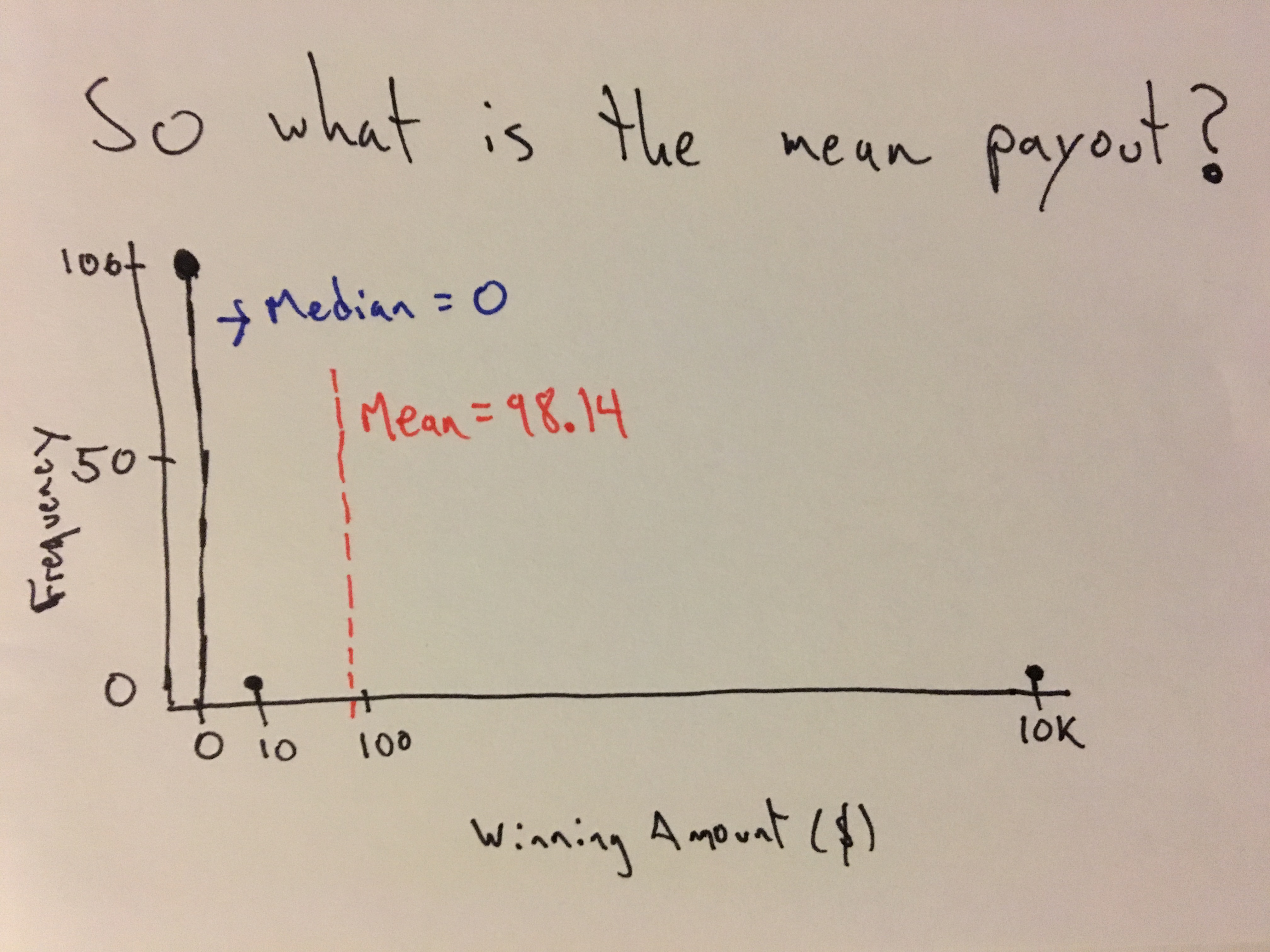# Pragmatic Stats: Mean# What is it?

The mean is the average of a sample or population of scores. To break it down one step further, it is the sum of scores divided by the number of scores in the sample or group. It is one of the primary measures of centrality in statistics.

# What is it used for?

It provides insight into what our data looks like. However it is a statistic (parameter in the case of a population) that is used in many different applications in statistics, machine learning, etc. I won’t dive into those right now but, in upcoming posts it will be referenced often.

# Symbols & Formulas

μ - Symbol Name (Greek Letter): Mu , Parameter Name: Population Mean
X̄ - Pronunciation: “X hat”, Statistic Name: Sample Mean

Population Mean
$$\begin{equation} \mu = {\Sigma X \over N} \end{equation}$$

Sample Mean
$$\begin{equation} \bar{X} = {\Sigma X \over n} \end{equation}$$

n - number of scores in a sample
N - number of scores in a population
X - individual score

# When Should I Use This?

#### Use:

It should be used to ‘get a sense of your data’. Outside of exploration of your data, it can also be used for application like mean filtering to try and eliminate noise from a signal and/or perform time series decomposition.

#### Don’t Use:

Not sure there is a blanket time not to use it (at least in the case of exploratory analysis). But there are some things you should know (see next section).

# Assumptions, Prerequisites, and Pitfalls

The mean is great for giving us an idea of what our data looks like….. but it does not tell the whole story. This is exactly why we have other measures of centrality like the median and measures of variation such as variance and standard deviation. In upcoming post I’ll dive into some of these.

# Example - Sans CodeThe mean gives us some information, but the following example shows how it can often be misleading. For example, we have a sample set of scratch off lottery ticket outcomes.If we only look at the mean we might think our chance of winning is pretty decent, but if we look at the median, we might think differently ….